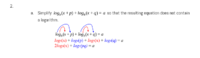# Simplifying a Log?

#### tkhunny

##### Moderator
Staff member
No. log(x+p) = log(x+p) No log simplification, there. This is not an application of the Distributive Property.

log(x+p) + log(x+q) = log((x+p)(x+q)) -- Turns addition outside the logarithm function into multiplication inside the logarithm function.

You did this part well: log(p) + log(q) = log(pq)...except that you never should have been here. Apply this concept consistently and from the beginning.

•knpoe03

#### Dr.Peterson

##### Elite Member
Hello!

I'm a bit confused by this question in my math homework. It instructs me to simplify out an equation so that the result does not contain a log. I've attempted to start this, but I'm really not sure if what I am doing is correct or even close to what I should be doing. I'd appreciate your help!

View attachment 23255
Changing it to not contain a logarithm means changing a logarithmic equation to an exponential equation.

•knpoe03

#### Subhotosh Khan

##### Super Moderator
Staff member
Hello!

I'm a bit confused by this question in my math homework. It instructs me to simplify out an equation so that the result does not contain a log. I've attempted to start this, but I'm really not sure if what I am doing is correct or even close to what I should be doing. I'd appreciate your help!

View attachment 23255Combine the two logs in the left-hand-side using properties of "log".

logb(x+p) + logb(x+q) $$\displaystyle \ \to \ \$$ logb[(x+p)*(x+q)]​

So now you have:

logb[(x+p)*(x+q)] = a​

Now eliminate "log" by using "exponential" (power) according to the definition of logarithm.

•knpoe03

#### knpoe03

##### New member
View attachment 23256
Combine the two logs in the left-hand-side using properties of "log".

logb(x+p) + logb(x+q) $$\displaystyle \ \to \ \$$ logb[(x+p)*(x+q)]​

So now you have:

logb[(x+p)*(x+q)] = a​

Now eliminate "log" by using "exponential" (power) according to the definition of logarithm.
So, to eliminate the log by using exponents, I would use b^n=m. This would change the equation to b^a=(x+q)(x+p). Is this correct?

•Subhotosh Khan

#### knpoe03

##### New member
Changing it to not contain a logarithm means changing a logarithmic equation to an exponential equation.
So to change it to an exponential function, I would use b^n=m. I got b^a=(x+p)(x+q). Is this correct? I'm not entirely familiar (or comfortable) with logs, so I appreciate any help you can offer.

#### knpoe03

##### New member
No. log(x+p) = log(x+p) No log simplification, there. This is not an application of the Distributive Property.

log(x+p) + log(x+q) = log((x+p)(x+q)) -- Turns addition outside the logarithm function into multiplication inside the logarithm function.

You did this part well: log(p) + log(q) = log(pq)...except that you never should have been here. Apply this concept consistently and from the beginning.
Thanks for your response! I now see where I went wrong.

#### Harry_the_cat

##### Elite Member
So to change it to an exponential function, I would use b^n=m. I got b^a=(x+p)(x+q). Is this correct? I'm not entirely familiar (or comfortable) with logs, so I appreciate any help you can offer.
Yes that's correct. Well done!

•knpoe03